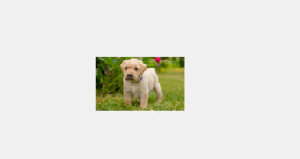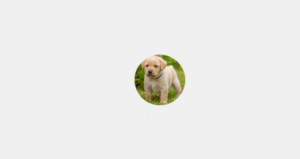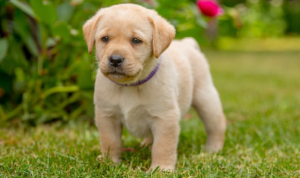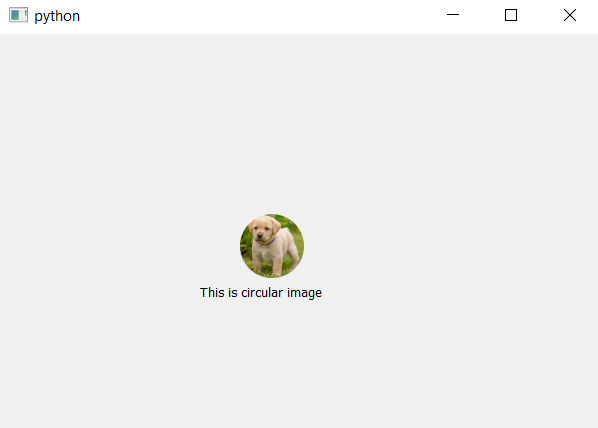# PyQt5 – How to create circular image from any image ?

In this article, we will see how to display only circular/round image from any image with any width and height i.eIn order to do so we have to do the following steps :

2. Crop image to make it square
3. Mask it and make circle from it using Painter
4. Convert it back to pixmap image

Code :

Below is the original image :`# importing libraries ` `from` `PyQt5.QtCore ``import` `*`  `from` `PyQt5.QtGui ``import` `*`  `from` `PyQt5.QtWidgets ``import` `*` ` `  `# function to alter image ` `def` `mask_image(imgdata, imgtype ``=``'png'``, size ``=` `64``): ` ` `  `    ``# Load image ` `    ``image ``=` `QImage.fromData(imgdata, imgtype) ` ` `  `    ``# convert image to 32-bit ARGB (adds an alpha ` `    ``# channel ie transparency factor): ` `    ``image.convertToFormat(QImage.Format_ARGB32) ` ` `  `    ``# Crop image to a square: ` `    ``imgsize ``=` `min``(image.width(), image.height()) ` `    ``rect ``=` `QRect( ` `        ``(image.width() ``-` `imgsize) ``/` `2``, ` `        ``(image.height() ``-` `imgsize) ``/` `2``, ` `        ``imgsize, ` `        ``imgsize, ` `     ``) ` `      `  `    ``image ``=` `image.copy(rect) ` ` `  `    ``# Create the output image with the same dimensions  ` `    ``# and an alpha channel and make it completely transparent: ` `    ``out_img ``=` `QImage(imgsize, imgsize, QImage.Format_ARGB32) ` `    ``out_img.fill(Qt.transparent) ` ` `  `    ``# Create a texture brush and paint a circle  ` `    ``# with the original image onto the output image: ` `    ``brush ``=` `QBrush(image) ` ` `  `    ``# Paint the output image ` `    ``painter ``=` `QPainter(out_img) ` `    ``painter.setBrush(brush) ` ` `  `    ``# Don't draw an outline ` `    ``painter.setPen(Qt.NoPen) ` ` `  `    ``# drawing circle ` `    ``painter.drawEllipse(``0``, ``0``, imgsize, imgsize) ` ` `  `    ``# closing painter event ` `    ``painter.end() ` ` `  `    ``# Convert the image to a pixmap and rescale it.  ` `    ``pr ``=` `QWindow().devicePixelRatio() ` `    ``pm ``=` `QPixmap.fromImage(out_img) ` `    ``pm.setDevicePixelRatio(pr) ` `    ``size ``*``=` `pr ` `    ``pm ``=` `pm.scaled(size, size, Qt.KeepAspectRatio,  ` `                               ``Qt.SmoothTransformation) ` ` `  `    ``# return back the pixmap data ` `    ``return` `pm ` ` `  ` `  `class` `Window(QWidget): ` `     `  `    ``"""Simple window that shows our masked image and text label."""` `    ``def` `__init__(``self``): ` `        ``super``().__init__() ` ` `  `        ``# setting up the geometry ` `        ``self``.setGeometry(``100``, ``100``, ``600``, ``400``) ` ` `  `        ``# image path ` `        ``imgpath ``=` `"image.png"` ` `  `        ``# loading image ` `        ``imgdata ``=` `open``(imgpath, ``'rb'``).read() ` ` `  `        ``# calling the function ` `        ``pixmap ``=` `mask_image(imgdata) ` ` `  `        ``# creating label ` `        ``self``.ilabel ``=` `QLabel(``self``) ` ` `  `        ``# putting image on label ` `        ``self``.ilabel.setPixmap(pixmap) ` ` `  `        ``# moving the label ` `        ``self``.ilabel.move(``240``, ``180``) ` ` `  `        ``# another label to put text ` `        ``self``.tlabel ``=` `QLabel(``'This is circular image'``, ``self``) ` `        ``self``.tlabel.move(``200``, ``250``) ` ` `  ` `  ` `  `# main function ` `if` `__name__ ``=``=` `'__main__'``: ` `    ``import` `sys ` `    ``from` `PyQt5.QtWidgets ``import` `QApplication ` ` `  `    ``# app created ` `    ``app ``=` `QApplication(sys.argv) ` `    ``w ``=` `Window() ` `    ``w.show() ` ` `  `    ``# begin the app ` `    ``sys.exit(app.exec_()) `

Output :My Personal Notes arrow_drop_upCheck out this Author's contributed articles.

If you like GeeksforGeeks and would like to contribute, you can also write an article using contribute.geeksforgeeks.org or mail your article to contribute@geeksforgeeks.org. See your article appearing on the GeeksforGeeks main page and help other Geeks.

Please Improve this article if you find anything incorrect by clicking on the "Improve Article" button below.

Article Tags :

Be the First to upvote.

Please write to us at contribute@geeksforgeeks.org to report any issue with the above content.